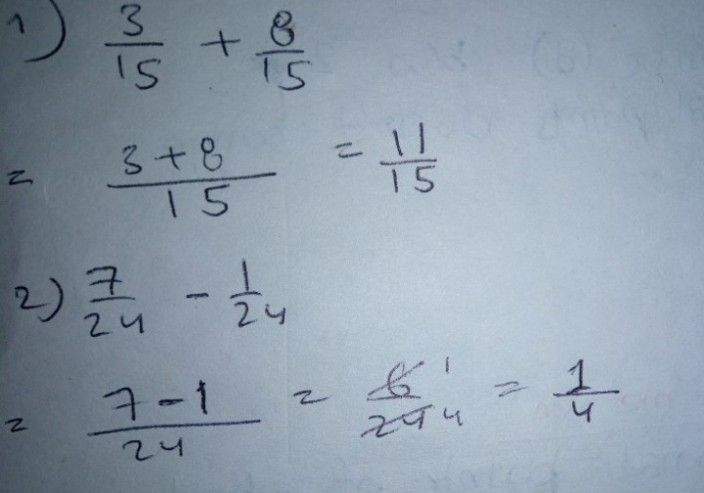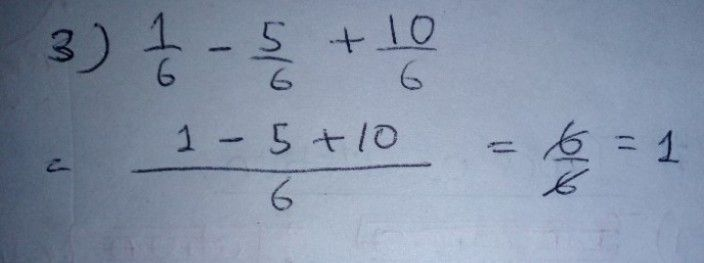Symbol
Problem$Dlt0c1lon0$ $Pon\left(orn$ the indicated operations and reduce your answers to the lowest Write your answers on a separate sheet of paper. $1$ $\dfrac {3} {15}+\dfrac {a} {15}$ $2$ $\dfrac {7} {24}-\dfrac {1} {24}$ $3.1$ $-$ $\dfrac {1} {6}-\dfrac {5} {6}+\dfrac {10} {6}$ $1ostlon9:$ What did $y$ you do to reduce the expressions in Activity $A7$ What do you call all the groups of fractions in Activity B? Why? Arrange the following steps of adding and subtracting similar fractions. Write a, b Numerators are added or subtracted and the common denominator to arrange them. The fractions are combined into one fraction. Common factor or factors of the numerator and denominator $slare$ out. The numerators and denominators are expressed into prime facto $2$
7th-9th grade
Algebra
Search count: 126
SolutionQanda teacher - missPreetiIf you have any doubts please do let me know dear. if you are satisfied with my answer please do LIKE and give a 5 STAR RATING and coins as THANK U GIFT..! ALL THE BEST..!!!If you have more questions then please ask through preffered match from my profileStudent
OKQanda teacher - missPreeti
To evaluate:- 1. Click on top right corner evaluate button. 2. Select 5 star 3. Click on gift coins + three times. 4. Click on DONE button on top right corner.